# What is the point of Gaussian elimination

## Gaussian algorithm or Gaussian method

You can learn here how to use the Gaussian algorithm (also known as the Gaussian algorithm or Gaussian elimination method). Let's look at this:

• A Explanation how to use the Gaussian method or the Gaussian algorithm.
• Examples how to use it to solve systems of equations.
• Tasks / exercises so that you can practice this yourself.
• A Video to linear systems of equations.
• A Question and answer area to the Gaussian elimination method.

Tip: The Gaussian method is one way of solving a linear system of equations. You can learn more methods in our main article under Solving systems of linear equations.

### Explanation of the Gaussian elimination process

In mathematics, equations are always solved. In some cases it happens that you have multiple equations with multiple variables (x, y, z, or other). These equations must be solved jointly. Something like that is called solving a (linear) system of equations.

One way of solving a system of equations is called the Gauss method. Other names for it are Gaussian algorithm or Gaussian elimination method. So we hold on:

Note:

The Gaussian elimination method is used to solve linear systems of equations. A number is to be found for each variable that correctly solves all equations.

The aim of the Gaussian method is to create a system of equations in which all variables are contained in the first row and one variable has been eliminated in each subsequent row below. The procedure is as follows:

1. Put all terms with variables on one side of the equation and only the numbers on the other side.
2. The same variables should appear one below the other in all equations.
3. Generate the same factors for all equations by multiplication or division (sign different but is OK).
4. Throw out a variable by adding or subtracting the equations.
5. Repeat this until there is only one variable left and calculate it.
6. Insert backwards to calculate all remaining variables.

Note: With the Gaussian method, many steps can be summarized very briefly. However, many beginners have problems understanding the calculation steps as a result. Everyone has to decide for themselves how many steps are necessary to solve.

For a better understanding, we will look at an example in the next section, which is calculated and explained in more detail.

Display:

### Example Gaussian elimination method simply explained

Let's take a closer look at the Gaussian elimination method.

Example 1: 3 equations with 3 unknowns

We have a system of linear equations with three equations and three unknowns. This should be solved with the Gaussian elimination method. How big are x, y and z? Give the amount of solution.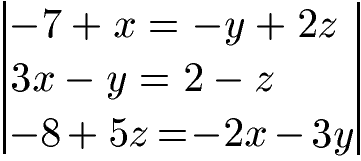Solution:

First, let's put all the variables on the left side of the equation and the pure numbers on the right side of the equation. The terms with x, y and z should stand one below the other.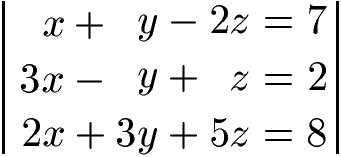First we want to eliminate x. By multiplying or dividing all equations, the same factors should be generated in all equations. The easiest way to do this is to generate 6x on each equation. So we multiply the first equation by 6, the second equation by 2 and we multiply the third equation by 3.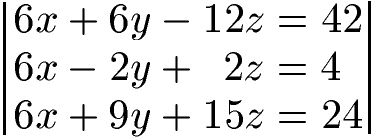Now we subtract:

• We take the top equation and subtract the middle equation from it. In front we get 6x - 6x = 0. Then 6y - (-2y) = 8y and -12z - 2z = -14z. On the right side 42 - 4 = 38.
• We take the top equation and subtract the bottom equation from it. In front we get 6x - 6x = 0. Then 6y - 9y = -3y. Also -12z -15z = -27z. On the right side 42 - 24 = 18.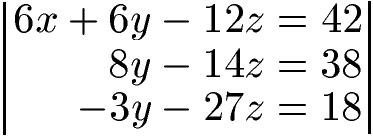With 8y -14z = 38 and -3y - 27z = 18 we still have two equations with two unknowns. Next, let's throw y out. To do this, we multiply the middle equation by 3 and the bottom equation by 8.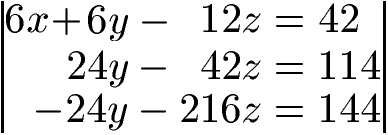We now add: the middle equation plus the lowest equation. We get 24y + (-24y) = 0. Also -42z + (-216z) = -258z. On the right hand side of the equation, we get 114 + 144 = 258.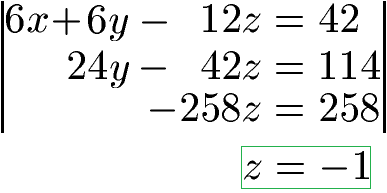With -258z = 258 we get z = -1 as the solution. We plug this into the middle equation 24y -42z = 114 and use it to calculate y = 3.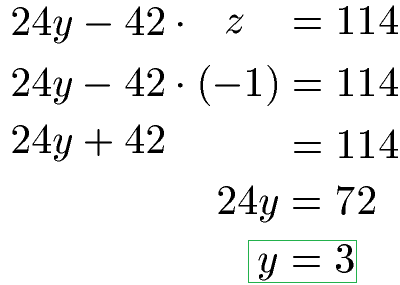With y and z we go into an equation with all variables and calculate x.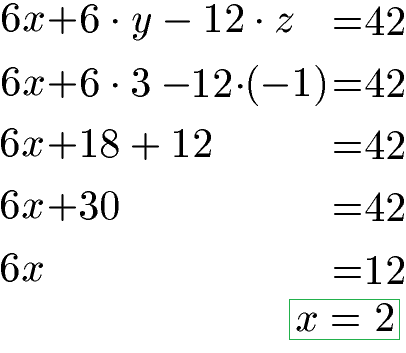We have calculated the solution. We get x = 2, y = 3 and z = -1.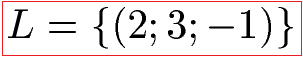Show:

### Solve LGS using the Gaussian method

The Gaussian elimination method is shown in the next video. An example is first simplified by using a notation as a matrix. The task is then solved using the Gaussian method. The next video is also from Youtube.com.

The equations in the example are:

• x + y + z = 6
• y + z = 5
• 2x - y + z = 3

Next video »

### Questions with answers about the Gaussian method

In this section we look at typical questions with answers about the Gaussian method.

Q: How can one still solve systems of linear equations?

A: Linear systems of equations cannot only be calculated using the Gaussian method. Alternatively, there is also the equation process, the substitution process, or the addition process or subtraction process. With a little time, you can learn by yourself whether the respective procedure is useful or not while working on the tasks.

Q: What other topics should I check out?

A: In addition to the linear systems of equations, there are also different types of equations. Have a look at this content: